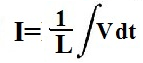﻿ Inductor Current Calculator ﻿# Inductor Current CalculatorVoltage, V V Inductance, L H (henries) mH (millihenries) µH (microhenries) nH (nanohenries) pH (picohenries) Current, I

Example Voltages To Enter
5sin(60t)
10cos(110t)
15sin(120t)

This Inductor Current Calculator calculates the current flowing through an inductor based on the voltage, V, across the inductor and the inductance, L, of the inductor.

The formula which calculates the inductor current based on these input parameters is I= 1/L∫Vdt, where I is equal to the current flowing through the inductor, L is equal to the inductance of the inductor, and V is equal to the voltage across the inductor. Many times, you will see the extended formula, I= I0 + 1/L∫Vdt. This formula takes into account any initial current that may be flowing across the inductor to begin with. However, for the sake of simplicity, we have made this calculator not accept initial conditions. If you have initial conditions, just simply add that value with the value that this calculator computes.

The integral of the voltage, V, really means that the sum of all the voltages are added up with respect to time.

The voltage can be a DC or AC value. If it is DC, a numerical value is entered into the calculator. If it is AC, the value will be a sine or a cosine waveform. A sine or cosine waveform replicates an AC signal which may be seen in real life such as the AC power which comes out of a wall outlet. So values such as sin(60t), 10sin(120t), 15cos(110t) can be entered into the calculator. And the resultant integral will be computed for it.

To use this calculator, a user simply enters the voltage, V, and inductance, L. The user can decide if s/he wants the answer computed in fractional form or decimal form. S/he then clicks the 'Calculate' button, and the resultant voltage value in unit amperes (A) is automatically computed and displayed.

Examples

What is the current flowing across an inductor if the voltage is 6sin(60t) and the inductance is 2H?

V= 1/L∫Vdt= (1/2C)∫(6sin(60t))= -(6/120)cos(60t) A

So the current flowing across the inductor is -(6/120)cos(60t) A in fractional form.
The same value is -0.05cos(60t) V in decimal form.

What is the current flowing across an inductor if the voltage is 5cos(60t) and the inductance is 5H?

V= 1/L∫Vdt= (1/5H)∫(5cos(60t))= (5/300)sin(60t) A

So the current flowing across the inductor is (5/300)cos(60t) A.

Related Resources

﻿